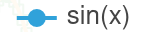# Combine legends of gv.Path and gv.Points

Hi,

I am plotting a “line” with gv.Path and I plot with the same data using gv.Points, to have Points overlayed on this line.

Here is the code :

``````    track_plot = gv.Points(track_gdf, vdims=["wind_speed (m/s)", "date"]).opts(
color="wind_speed (m/s)", cmap=high_cmap, size=10, tools=["hover"], clim=(0, 80), line_color="black",
line_width=1.5)

...

track_path_plot = gv.Path(track_path, vdims=["wind_speed (m/s)", "date"],
label="Cyclone ATCF interpolated track").opts(
color="black", tools=["hover"], line_width=3, show_legend=True)
``````

Plotting the data works fine.

I would like that the legend indicates something like this :Is it possible ?

Thanks !

If you set `label="Cyclone ATCF interpolated track"` for track_plot it should work

1 Like

Oh great it works ! I was sure I tried this before without success. Thanks for your help !